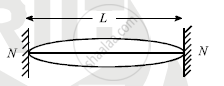# Derive an Expression for Frequency in Fundamental Mode - Physics

A wire of density ‘ρ’ and Young’s modulus ‘Y’ is stretched between two rigid supports separated by a distance ‘L’ under tension ‘T’. Derive an expression for its frequency in fundamental mode. Hence show that n=1/(2L)sqrt((Yl)/(rhoL)) where symbols have their usual meanings

#### Solution

onsider a string of lenght ‘L’. Let ‘m’ be the mass per unit length of the stirng ‘T’ be tension in the string.

If transveres wave is produced in the string, the velocity of the wave is given by v=sqrtT/m""....(1)If the string is plucked in middle, two incident and reflected wave will produce stationary wave. The string will vibrate in different mode which is called mode of vibrations.

The simplest mode of vibration is as shown in figure, which is called fundamental mode of vibration. Here two nodes and one antinode is formed. Let λ and n be corresponding wavelength and frequency

∴ L=λ/2    ∴λ=2L     ∴v=nλ=n2L

:."From equation (i)"n(2L)=sqrt(T/m)

:.n=1/(2L)sqrt(T/m)" ....(2)"

This is called fundamental frequency

Young’s modulus

Y=(TL)/(Al)

T=(YAl)/L, m=M/L=(Vrho)/L

=(ALrho)/L=Arho

:."from equation (2) "n=1/(2L)sqrt(((YAl)/L)/(Arho))

n=1/(2L)sqrt((Yl)/(AL))

Concept: Reflection of Transverse and Longitudinal Waves
Is there an error in this question or solution?
2013-2014 (March)

Share